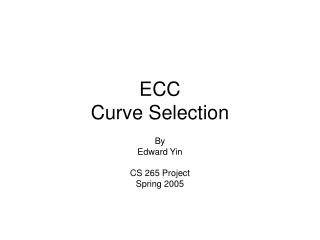DownloadDownload PresentationECC Curve Selection

# ECC Curve Selection

Download Presentation## ECC Curve Selection

- - - - - - - - - - - - - - - - - - - - - - - - - - - E N D - - - - - - - - - - - - - - - - - - - - - - - - - - -
##### Presentation Transcript

1. ECCCurve Selection By Edward Yin CS 265 Project Spring 2005

2. Why ECC? Key Size, Speed, and Scalability NIST guidelines for equivalent strengths:

3. ECC Basics • Prime: GF(p) Y2 = X3 + aX + b with 4a3 + 27b2≠ 0 • Binary: GF(2m) Y2 + XY = X3 + aX2 + b with b ≠ 0 An “elliptic curve” means points on the curve plus the point at infinity. Private: integer k Public: a, b, point P, point Q=kP

4. Discrete Logs • Discrete Log Problem (DLP) • Given p, g, and y, find x such thatgx = y (mod p). • ECDLP • Given P, Q, find k such that kP = Q. • Diffie-Hellman Problem (DHP) • Given p, g, ga, gb, find gab (mod p). • ECDHP • Given P, sP, tP, find stP.

5. DLP and ECDLP

6. Known Attacks Best general attack is the Pollard rho method, taking O(n1/2) curve additions, where n is the order of the base point P (smallest positive integer such that nP = 0). Shortcuts: • The Pohlig-Hellman algorithm reduces the size of the problem. • ECDLP reduced to ECDLP modulo each prime factor of n • ECDLP for anomalous curves in a prime field is solvable in polynomial time. • Prime-field-anomalous if group order = field order = n • ECDLP for some curves (e.g. supersingular curves) is solvable in subexponential time • MOV reduction possible if (field order)k = 1 (mod n) for some k

7. Avoiding Weak Curves • #E(GF(q)) = hn with large prime n, small h and nP=0. • #E(GF(q)) ≠ q. • The order n of point P should not divide qk-1 for all 1 ≤ k ≤ C, C≥20 in practice.

8. Approaches to Curve Selection • Choose the group order first • Use the Complex Multiplication method (CM) • Construct curve from another known curve • Choose a random curve • Count points with Schoof’s algorithm or the Schoof-Elkies-Atkin (SEA) algorithm • Use a published curve Algorithms: see e.g. IEEE P1363 Annex A. Implementation: see e.g. MIRACL at http://indigo.ie/~mscott/